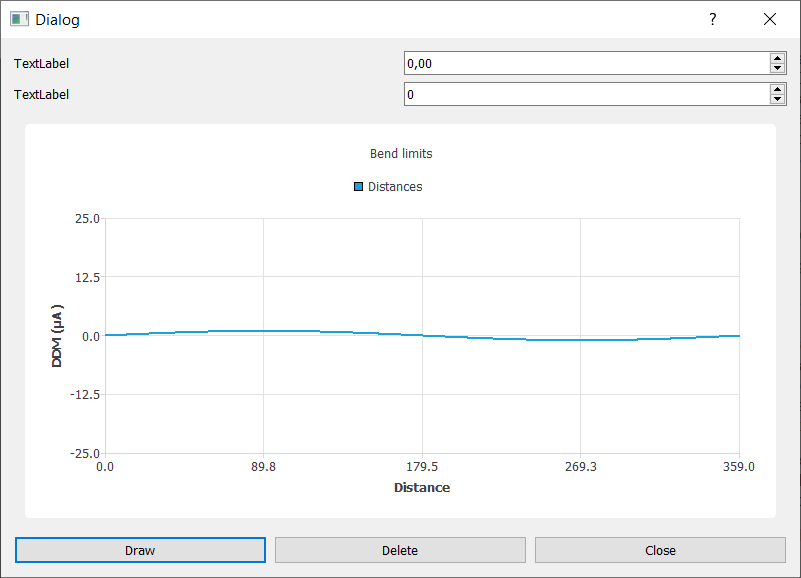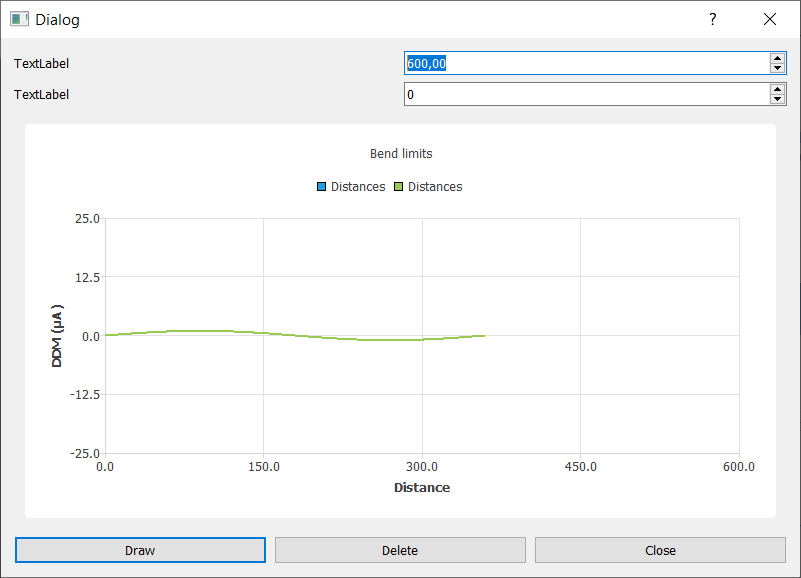# QChart setting axis

• Hi,
I'm a beginner in Qt and have some trouble with the QChart widget.

Here is my code:
In the constructor:

``````ui->setupUi(this);
m_chart = ui->graphView->chart();

m_xAxis = new QValueAxis;
m_xAxis->setMax(1200.0);
m_xAxis->setMin(0.0);
m_xAxis->setTitleText("Distance");

m_yAxis = new QValueAxis;
m_yAxis->setMax(25.0);
m_yAxis->setMin(-25.0);
m_yAxis->setTitleText("DDM (µA)");
m_chart->setTitle("Bend limits");
``````

A pushButton with the following code:

``````QLineSeries *series = new QLineSeries();
m_series.append(series);
QList<QPointF> data;
for (int i = 0; i < 360; i++)
{
}
series->setName("Distances");
series->attachAxis(m_xAxis);
series->attachAxis(m_yAxis);
``````

A doubleSpinBox for changing the Axis range with the following code:

``````double test = ui->doubleSpinBox->value();
m_xAxis->setRange(0.0, test);
``````

The problem is that each time I change the m_xAxis range a new plot is added.

First click on Draw buttonSetting X max value to 600What is the reason for this behavior and how can I get rid of it?
Thank you